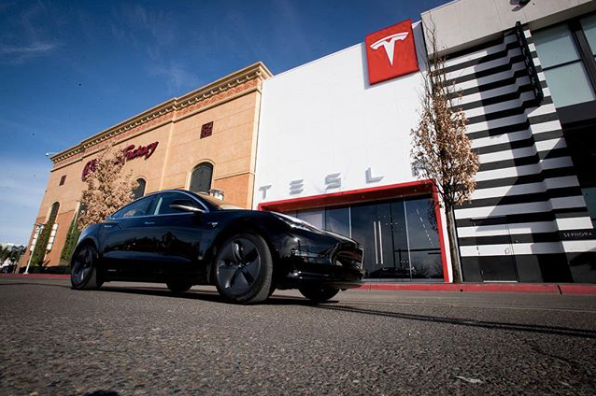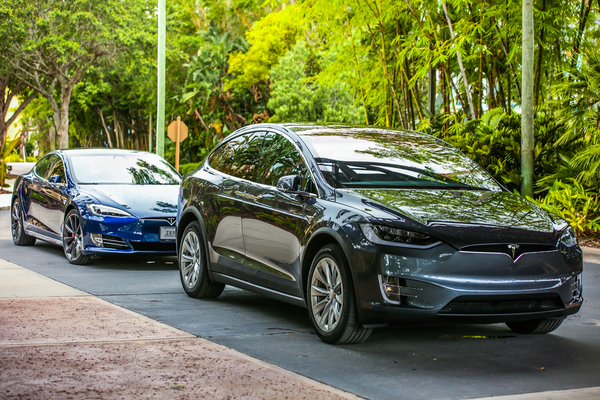# Tesla Model 3 profit target is 5x higher than the average vehicle from Ford

Posted on February 17, 2018 by Matt Pressman

It's remarkable that Tesla rocketed from its IPO to surpassing Ford in value in just 7 years. Tesla's prowess on Wall Street have left many investors miffed. After all, Ford recently announced 2017 sales of over 6.6 million cars worldwide. Meanwhile Tesla barely cracked sales of 100,000 cars. How could an industry behemoth like Ford be overtaken by this younger, smaller Silicon Valley start-up?Above: Can Tesla really outperform Ford? (Image: Petrolhead)

Dave Lee at Tesla Weekly has some fascinating observations on this front. Lee notes, "There appears to be a narrative amongst Tesla skeptics where the quantity of cars Tesla produces is compared to major automakers [like Ford] to minimize Tesla’s achievements... I’ll dive into this and show why the narrative is incorrect."

Looking at Ford's recently filed 2017 annual report, Lee reports that Ford sold: "6,607,000 vehicles at wholesale throughout the world. Ford’s 2017 automotive revenue was 145.6B, which means that the average revenue per vehicle was roughly \$22,000. Ford’s gross margin on automotive in 2017 was roughly 10%, and their profit margin (before taxes) was roughly 5%."

Putting this in perspective: "For an average priced \$22,000 car the Ford sells, their gross margin is \$2,200 and their profit margin is \$1,100. On average Ford makes \$1,100 per vehicle."Above: A look at the new Tesla Model 3 (Instagram: washingtonsq)

On the other hand, "let’s look at Tesla and the Model 3. Tesla is aiming for 25% gross margin on the Model 3 and mid-teens profit margin (let’s say 14%). The average price of the Model 3 is projected at around \$42,000... [so] the average gross margin on a Model 3 would be \$10,500 and profit margin would be \$5,880.  Compared to Ford’s average vehicle profit margin of \$1,100, the Model 3 would be 5x as profitable."

Lee continues, "In other words, one Model 3 is worth (in terms of profits) the equivalent of 5 Ford vehicles. So, if Tesla can sell 500,000 Model 3 and 500,000 Model Y (their small SUV due in 2020) annually, that would be 1M vehicles at an average of 5x the profitability of Ford’s vehicles. So the equivalent would be 5M Ford vehicles."

Taking it one step further, "Let’s add in the Model S/X to the mix." Lee points to historical data on Tesla's margins for Model S and X and extrapolates: "Profit margin on each S/X would be \$16,200 (if we assumed a average sale price of \$90k). That’s almost 15x as profitable as the average Ford vehicle. So, 100,000 Model S/X would be the equivalent of 1.5M Ford vehicles in terms of profit. Combine 1M Model 3/Y and 100k S/X and you have the equivalent of 6.5M vehicles from Ford."Above: Tesla's Model X and Model S (Image: EVANNEX)

"Let that sink in," Lee concludes. "If Tesla can achieve what they’re aiming for, then just 1.1M of their vehicles would produce the same profit as 6.5M vehicles from Ford. And Tesla would just be getting started."

===

Source: Tesla Weekly

Posted in Ford, Tesla, tesla news, TSLA

Next